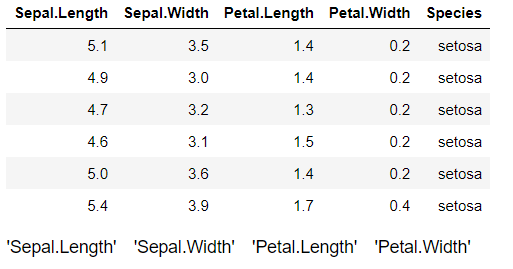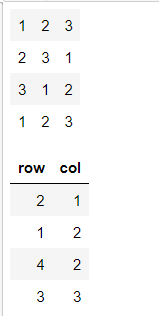# which() Function in R

• Last Updated : 23 Aug, 2021

which() function in R Programming Language is used to return the position of the specified values in the logical vector.

Syntax: which(x, arr.ind, useNames)

Parameters: This function accepts some parameters which are illustrated below:

• X: This is the specified input logical vector
• Arr.ind: This parameter returns the array indices if x is an array.
• useNames: This parameter says the dimension names of an array.

Return value: This function returns the position of the specified values in the logical vector.

### Example 1: Which() function applying tothe alphabet

In the below example, which() function returns the alphabetical position of the specified letter. For example, a is the first letter in the alphabet sequence that’s why 1 is returned and z is the last letter in the sequence so 26 is returned.

## R

 `# R program to illustrate``# which() function`` ` `# Calling the which function``# to return alphabetical position``# of the given alphabet``which``(``letters` `== ``"a"``)``which``(``letters` `== ``"d"``)``which``(``letters` `== ``"z"``)``which``(``letters` `== ``"p"``)``which``(``letters` `== ``"g"``)`

Output :

``` 1
 4
 26
 16
 7```

### Example 2:  which() function with vectors

In the below example, the position of some elements of the specified vector is being returned which the help of which() function.

## R

 `# R program to illustrate``# which() function`` ` `# Creating a vector of some elements``vector <- ``c``(3, 5, 1, 6, 12, 4)`` ` `# Getting the position of element 12``# in the above vector``which``(vector == 12)`` ` `# Getting the position of element 1``# in the above vector``which``(vector == 1)`` ` `# Getting the position of element 6``# in the above vector``which``(vector == 6)`` ` `# Getting the position of elements``# those are greater than 5``which``(vector > 5)`

Output:

``` 5
 3
 4
 4 5```

### Example 3: which() function with dataframe

In the below example, which() function is used to find the columns in a data frame with numeric values.

An Iris data set is used as a data frame that contains 4 columns for numerical values and 1 column for categorical values i.e., Species. The which() function find the columns name from the data set that contain numeric values.

## R

 `# Considering “Iris” dataset``data_set <- datasets::iris`` ` `# Printing the Iris dataset values``# along with its 5 columns out of which``# 4 columns are numerical and 1 is categorical``# (Species)``head``(data_set)`` ` `# Calling the which() function over``# the above specified data set that ``# returns the columns with numeric values``Result <- ``which``(``sapply``(data_set, is.numeric))`` ` `# Printing the columns with numeric values``colnames``(data_set)[Result]`

Output:### Example 4: which() function with the matrix

In the below example, which() function is used to find the position of an element in the specified matrix.

Here the position of value 2 in the specified matrix is being calculated.

## R

 `# Creating a matrix of 3 columns and 4 rows``Matrix <- ``matrix``(``rep``(``c``(1, 2, 3), 4), nrow = 4)`` ` `# Printing the entire matrix with its values``Matrix`` ` `# Calling the which() function to find the ``# position of value 2 in the above matrix``which``(Matrix == 2, arr.ind = T)`

Output:My Personal Notes arrow_drop_up